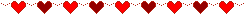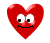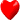Elementary Algebra Assignment #8Solve the following word problems:1. Twice a number increased by 7 is the same as 4 more than the number. What is the number? 2. The length of a rectangle is 2 more than 3 times the width. The perimeter is 44 inches. Find the width. 3. Ed jogged 4 times as many miles as Jamie, while Nick jogged 2 miles less than Jamie. If the three ran a total of 16 miles, how far did Ed go? 4. The length of a rectangle is 4 feet less than twice the width. Find the dimensions of the rectangle if the perimeter is 232 feet. 5. Jon earned \$230 more per month than Lori. If their monthly salaries total \$5,280, what amount does each earn? 6. There are 70 students registered in three algebra classes. There are twice as many students in the 10am class as the 8am class and there are 10 more students in the 12:00 class than in the 8:00 class. How many students are in each class? 7. The sum of three consecutive integers is 69. What is the largest interger?All Contents Copyright©1997-2017 by Ellen Freedman, All Rights Reserved.